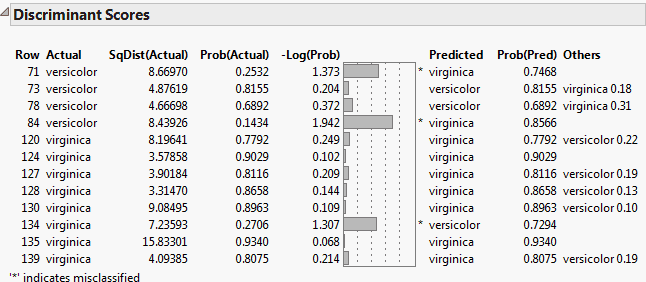Publication date: 08/13/2020

## Discriminant Scores

The Discriminant Scores report provides the predicted classification of each observation and supporting information.

Row

Row of the observation in the data table.

Actual

Classification of the observation as given in the data table.

SqDist(Actual)

Value of the saved formula SqDist[<level>] for the classification of the observation given in the data table. See Score Options.

Note: Due to an offset term in the formula, SqDist(Actual) can be negative.

Prob(Actual)

Estimated probability of the observation’s actual classification.

-Log(Prob)

Negative of the log of Prob(Actual). Large values of this negative log-likelihood identify observations that are poorly predicted in terms of membership in their actual categories.

A plot of -Log(Prob) appears to the right of the -Log(Prob) values. A large bar indicates a poor prediction. An asterisk(*) indicates observations that are misclassified.

If you are using a validation or a test set, observations in the validation set are marked with a “v” and those in the test set are marked with a “t”.

Predicted

Predicted classification of the observation. The predicted classification is the category with the highest predicted probability of membership.

Prob(Pred)

Estimated probability of the observation’s predicted classification.

Others

Lists other categories, if they exist, that have a predicted probability that exceeds 0.1.

Figure 5.11 shows the Discriminant Scores report for the Iris.jmp sample data table using the Linear discriminant method. The option Score Options > Show Interesting Rows Only option is selected, showing only misclassified rows or rows with predicted probabilities between 0.05 and 0.95.

Figure 5.11 Show Interesting Rows Only